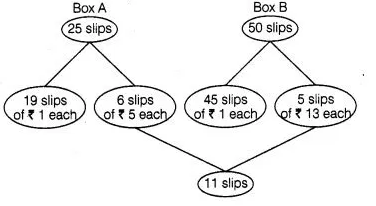# Box A contains 25 slips of which 19 are marked

Question:

Box A contains 25 slips of which 19 are marked  ₹ 1 and other are marked  ₹ 5 each. Box B contains 50 slips of which 45 are marked  ₹ 1 each and

others are marked  ₹ 13 each. Slips of both boxes are poured into a third box and resuffled. A slip is drawn at random. What is the probability that it is

marked other than  ₹ 1?

Solution:

Total number of slips in a box, n(S) = 25 + 50 = 75Erom the chart it is clear that, there are 11 slips which are marked other than $₹ 1$.

$\therefore \quad$ Required probability $=\frac{\text { Number of slips other than } ₹ 1}{\text { Total number of slips }}=\frac{11}{75}$